• 一维热传导方程热源反问题基于最小二乘法的正则化方法研究论文
• MATLAB编辑一维热传导方程的模拟程序 求解下列热传导问题 程序 function heat_conduction) %一维齐次热传导方程 options={'空间杆长L'空间点数N' '时间点数M'扩散系数alfa'稳定条件的值lambda(取值必须小于0.5,};...
• 学过计算流体力学的朋友，在控制方程数学性质方面，可能会遇到控制方程类型证明问题，例如：一维热传导方程、拉普拉斯方程、一维波动方程推导，建议多从克莱默法则入手，构造方程组，求解特征线。 ...
学过计算流体力学的朋友，在控制方程数学性质方面，可能会遇到控制方程类型证明问题，例如：一维热传导方程、拉普拉斯方程、一维波动方程推导，建议多从克莱默法则入手，构造方程组，求解特征线。二阶的导数部分，采用一阶导数替换，令,再结合全微分方程即可。

展开全文•MATLAB
• 求解下列热传导问题: 2T 1 T 门 c , 2 0 0 z L z t T乙0 1 z2 T 0,t 1, T L,t 0 L 1, 1 程序 fun ctio n heat_c on ductio n) % 一维齐次热传导方程 稳定条options={'空间杆长L'空间点数N'时间点数M'扩散系数alfa' ...
• MATLAB 编辑 一维 热传 导方程的模拟程序 求解下列热传导问题: 2T z2 T 0,t 1, T L,t L 1, 程序: fun ctio n heat_c on ductio n) % 一维齐次热传导方程 稳定条options={'空间杆长L'空间点数N'时间点数M'扩散系数...
• 含matlab程序，个人感觉很有帮助，在研究传热学的可以下来看看 含matlab程序，个人感觉很有帮助，在研究传热学的可以下来看看matlab程序
• 一维热传导方程一维热传导方程一维热传导方程一维热传导方程一维热传导方程
• 根据激光热源在生物组织中热传导的实际情况 ,提出一维热传导方程的一类边界条件和初始条件。采用数学物理方程定解求法分别得出有血流散热和无血流散热两种情况下一维瞬态温度时空分布的解析函数表达式 ,适合于生物...
• 热传导方程在地下水流动数值模拟、油藏数值模拟等工程计算中有着广泛应用，其并行实现是加速问题求解速度、提高问题求解规模的重要...因此，采用BJACOBI预处理技术的CG算法是种较好的求解三维热传导模型的并行方案。线性方程组 共轭梯度算法
• 通过偏微分方程描述了二无扩散热传导现象。基于有限容积法推导了该方程的离散代数方程组，针对恒定热流强度、恒定温度、对流换热和绝热这四种不同边界条件，分别讨论了热传导代数方程的离散系数和源项。通过MATLAB...
• pizh12thu：Python数值优化：使用Euler法求解二维热传导方程1​zhuanlan.zhihu.com移动热源移动热源在焊接分析、3D增材制造等瞬态分析中十分常见。先前计算式中给了恒定常数的热源 ,由于Numpy的广播机制， 与矩阵...上一篇实践了简单的二维热传导抛物线方程求解，对于简单的热源和等温边界条件进行了模拟，下面来探究一些更有趣的现象。pizh12thu：Python数值优化：使用Euler法求解二维热传导方程1​zhuanlan.zhihu.com移动热源移动热源在焊接分析、3D增材制造等瞬态分析中十分常见。先前计算式中给了恒定常数的热源,由于Numpy的广播机制，与矩阵进行相加操作时会被扩展成和U形状相同的矩阵进行运算，为了控制热源的移动，需要对矩阵形式的热源进行操作。U = U + rx*np.dot(U,A) + ry*np.dot(B,U) + heat*ft在Python代码中实现，指定一个具有空间位置的热源import numpy as np
import time
import matplotlib.pyplot as plt

if __name__ == "__main__":
a = 1
D = np.array([0,15,0,15])
Mx = 150
My = 150
T,Tn = 20,5
Nt = 20000

dx = (D-D)/Mx
dy = (D-D)/My
dt = T/Nt
Frame = 100
fq = 10
u0 = 20
u_env = 20

### 初始化U、A、B矩阵，各点初始温度u0
U = u0*np.ones((My+1,Mx+1))
# x方向二阶导系数矩阵A
A = (-2)*np.eye(Mx+1,k=0) + (1)*np.eye(Mx+1,k=-1) + (1)*np.eye(Mx+1,k=1)
# y方向二阶导系数矩阵B
B = (-2)*np.eye(My+1,k=0) + (1)*np.eye(My+1,k=-1) + (1)*np.eye(My+1,k=1)

#用网格化映射矩阵坐标信息
x=np.linspace(D,D,U.shape)
y=np.linspace(D,D,U.shape)
X,Y = np.meshgrid(x,y)
x0,y0 = 0,7 #热源中心位置

### 按时间增量逐次计算
for k in range(Nt+1):
tt = k*dt
#热源沿着X方向移动
x0 += 2*dt
Qv = 80*fq*np.exp(-8*((X-x0)**2+(Y-y0)**2))
# solve inside nodes
U = U + rx*np.dot(U,A) + ry*np.dot(B,U) + Qv*dt
# solve boundary nodes
U[:,0] = U[:,-1] = u_env
U[0,:] = U[-1,:] = u_env 用等温云图显示一下计算结果        if k%Frame == 0:
end = time.time()
print('T = {:.3f} s  process time = {:.1f}'.format(tt,end-start))
showcontourf(U,D,vmax=120)

def showcontourf(mat,D,cmap=plt.cm.get_cmap('jet'),fsize=(12,12),vmin=0, vmax=100):
plt.clf()
levels = np.arange(vmin,vmax,1)
x=np.linspace(D,D,mat.shape)
y=np.linspace(D,D,mat.shape)
X,Y=np.meshgrid(x,y)
z_max = np.max(mat)
i_max,j_max = np.where(mat==z_max), np.where(mat==z_max)
show_max = "U_max: {:.1f}".format(z_max)
plt.plot(x[j_max],y[i_max],'go')
plt.contourf(X, Y, mat, 100, cmap = cmap, origin = 'lower', levels = levels)
plt.annotate(show_max,xy=(x[j_max],y[i_max]),xytext=(x[j_max],y[i_max]),fontsize=14)
plt.colorbar()
plt.xlabel('x', fontsize=20)
plt.ylabel('y', fontsize=20)
plt.axis('equal')
plt.draw()
plt.pause(0.001)
plt.clf()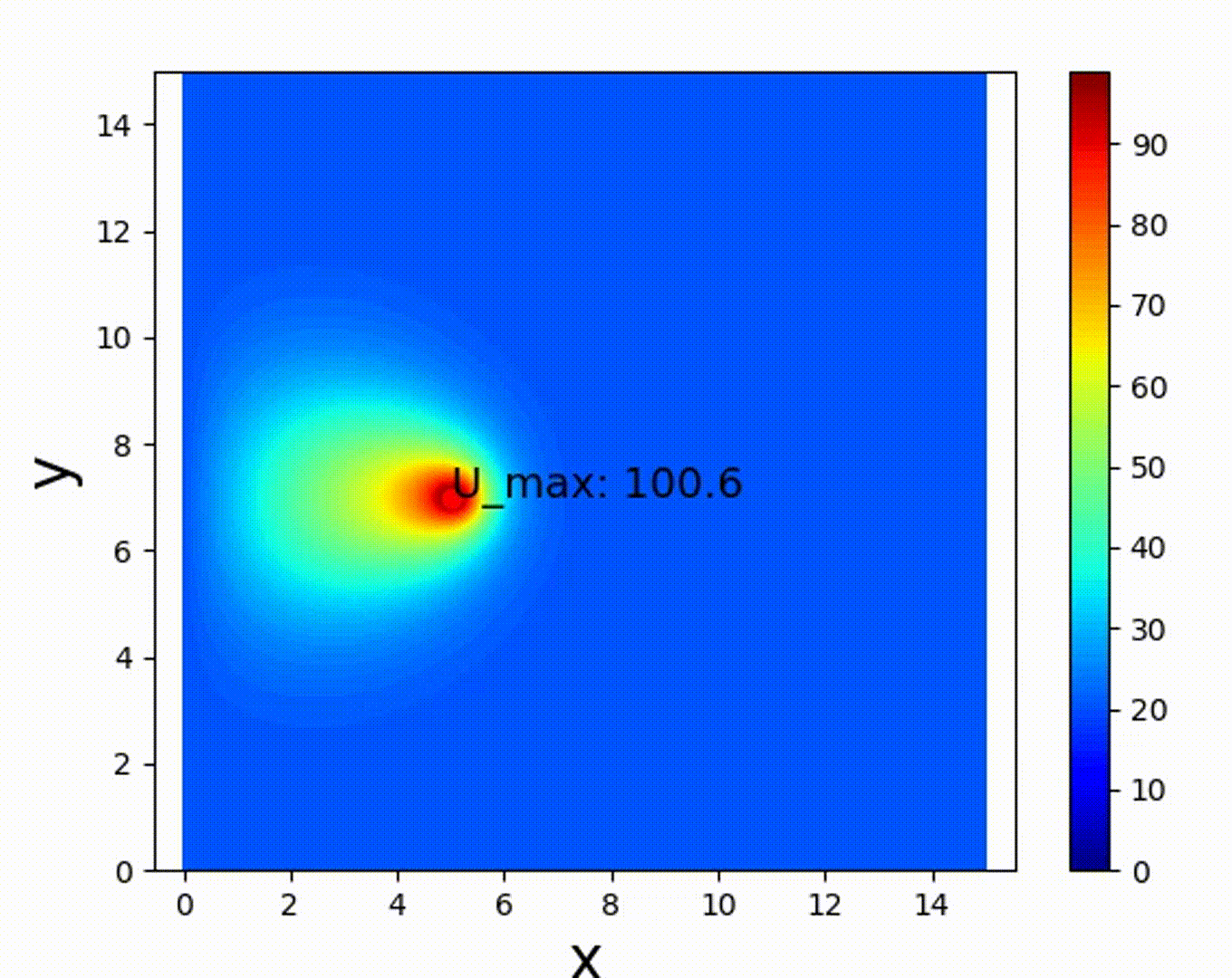移动内热源的实现Neumann边界条件可以看到热源向右匀速移动的效果，但是左右边界上似乎有点奇怪，边界没有受到热源的影响。这是因为我们给定了边界上的节点温度，即Dirichlet边界条件，边界温度与环境温度始终相同，那么如何处理热流边界条件呢        # solve boundary nodes
U[:,0] = U[:,-1] = u_env
U[0,:] = U[-1,:] = u_env 热流边界条件是第二类边界条件即Neumann边界条件，给定边界节点处的一阶导（斜率）同样采用差分格式离散化，则有通常边界给定对流换热系数，这样就可以建立热流平衡方程，使边界点内外两边的温度梯度达到平衡，即这样边界温度值就可以根据环境温度和内部节点温度的值加权平均求得，代表导热系数，表示边界处的对流换热系数，系数就反映了热传导和对流的相对大小，也决定了边界节点温度更接近内部还是外部温度。假定环境温度保持不变，边界均为对流散热边界，则有将 solve boundary nodes 部分改为如下形式        # solve boundary nodes
ch = 0.1
U[:,0] = (u_env + ch*U[:,1]/dx)/(1+ch/dx)
U[:,-1] = (u_env + ch*U[:,-2]/dx)/(1+ch/dx)
U[0,:] = (u_env + ch*U[1,:]/dy)/(1+ch/dy)
U[-1,:] = (u_env + ch*U[-2,:]/dy)/(1+ch/dy)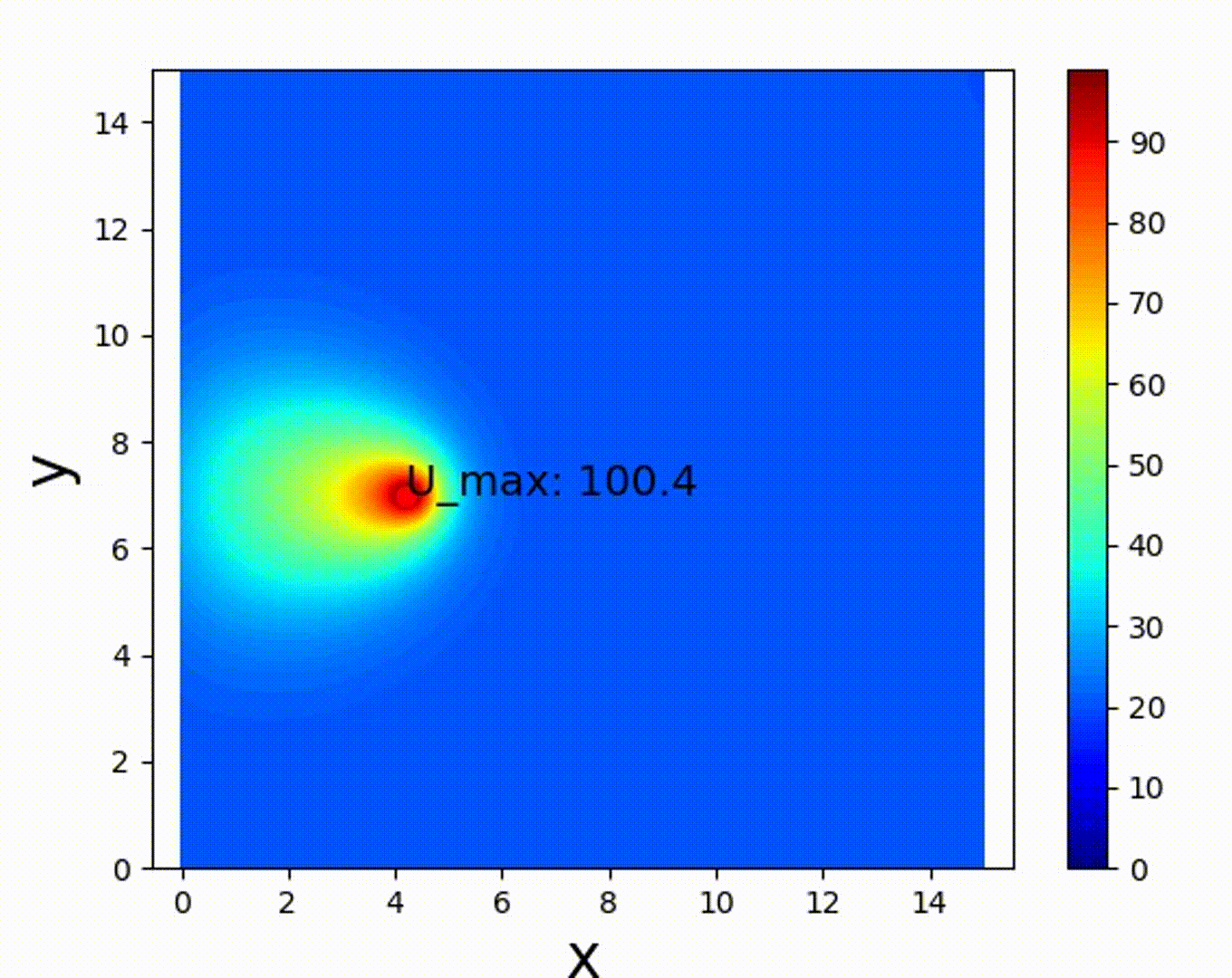对流换热边界条件，边界热量流出速度下降如果，，那么边界节点温度将等于环境温度，与等温边界结果相同。如果，，那么将没有热量可以从边界流出，此时就形成了绝热边界条件，边界温度等于内部相邻节点温度，结果如下图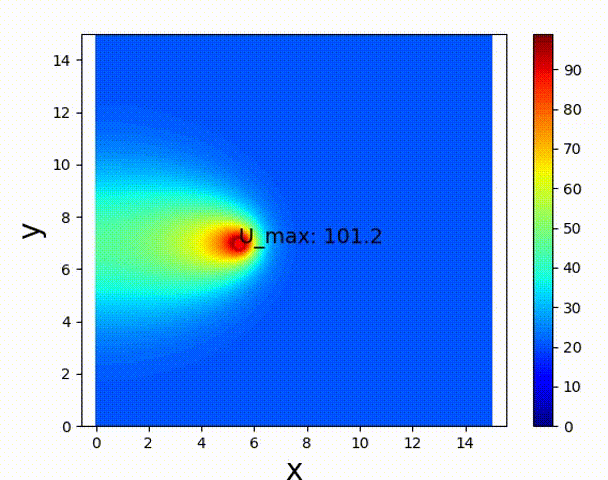绝热边界条件，等温线垂直于左右边界边界形状如果边界并非矩形，该如何用矩阵处理呢？关键在于确定矩阵中哪些节点是边界节点，可以使用Python中的Boolean逻辑矩阵。这里构造一个半径为7的圆形边界，用plt.imshow()来显示Boolean矩阵    x=np.linspace(D,D,U.shape)
y=np.linspace(D,D,U.shape)
X,Y = np.meshgrid(x,y)

Mat_e = (X-7.5)**2 + (Y-7.5)**2 >= 7**2
Mat_b = abs(((X-7.5)**2 + (Y-7.5)**2)**0.5 - 7) <= 0.5*min(dx,dy)
plt.imshow(Mat_b, cmap=plt.cm.get_cmap('jet'), origin = 'lower',vmin=0, vmax=1)
plt.show()Mat_e代表外部环境，Mat_b代表边界。离散的边界点不可能完全在解析曲线上，只要节点到边界曲线（圆周）的距离小于半个单元长度，就认为是边界节点。元素值为Ture的地方显示红色，False显示蓝色，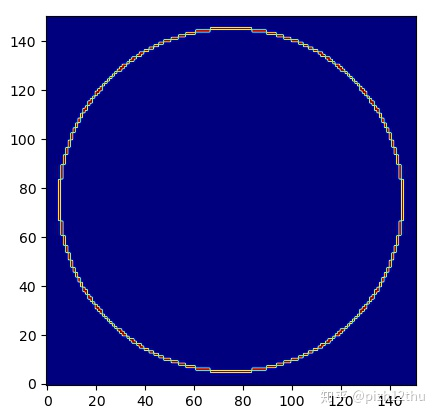边界节点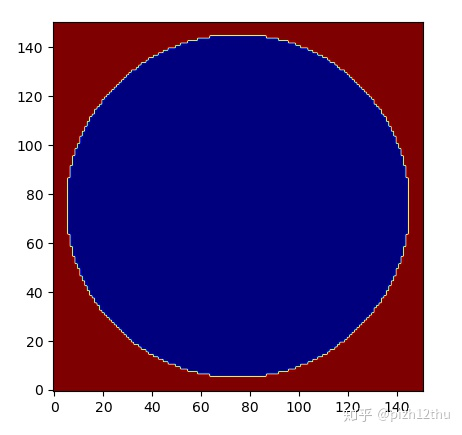外部节点求解边界时令外部节点温度等于环境温度，边界节点采用等效的热流边界，即以内部节点温度的最小值作为梯度参考，省略了计算法向的步骤。        # solve boundary nodes
ch = 0.1e6
U[Mat_e] = u_env
U[Mat_b] = (u_env + ch*np.min(U[~(Mat_e+Mat_b)])/dx)/(1+ch/dx)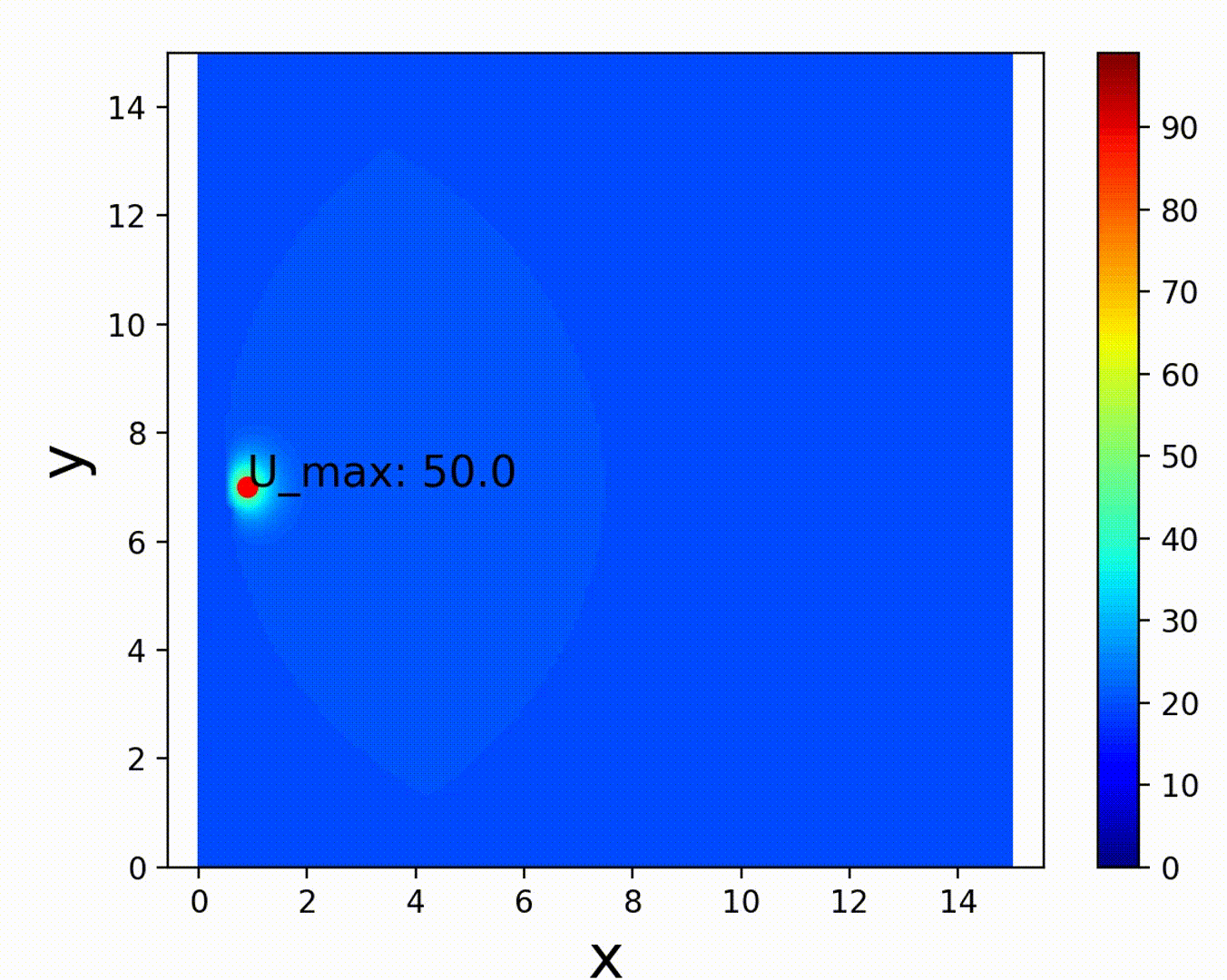可以看到变成了圆形边界，但温差太小，分界不是很明显。为了使结果表达更清晰，可以让外部节点不显示呢，Python中可以通过将对应位置的点赋值为np.nan实现#在函数showcontourf增加下列语句，且多传入一个参数M_e
def showcontourf(mat,D,M_e,cmap=plt.cm.get_cmap('jet'),fsize=(12,12),vmin=0, vmax=100):
...
...
Z = mat.copy()
Z[M_e] = np.nan
plt.contourf(X, Y, Z, 100, cmap = cmap, origin = 'lower', levels = levels)

#在main函数中,传入外节点Boolean矩阵
showcontourf(U,D,Mat_e,vmax=100)注意这里需要将mat复制一份（mat.copy()）再进行赋值操作，否则将会改变原数组mat，后续计算无法进行。显示结果如下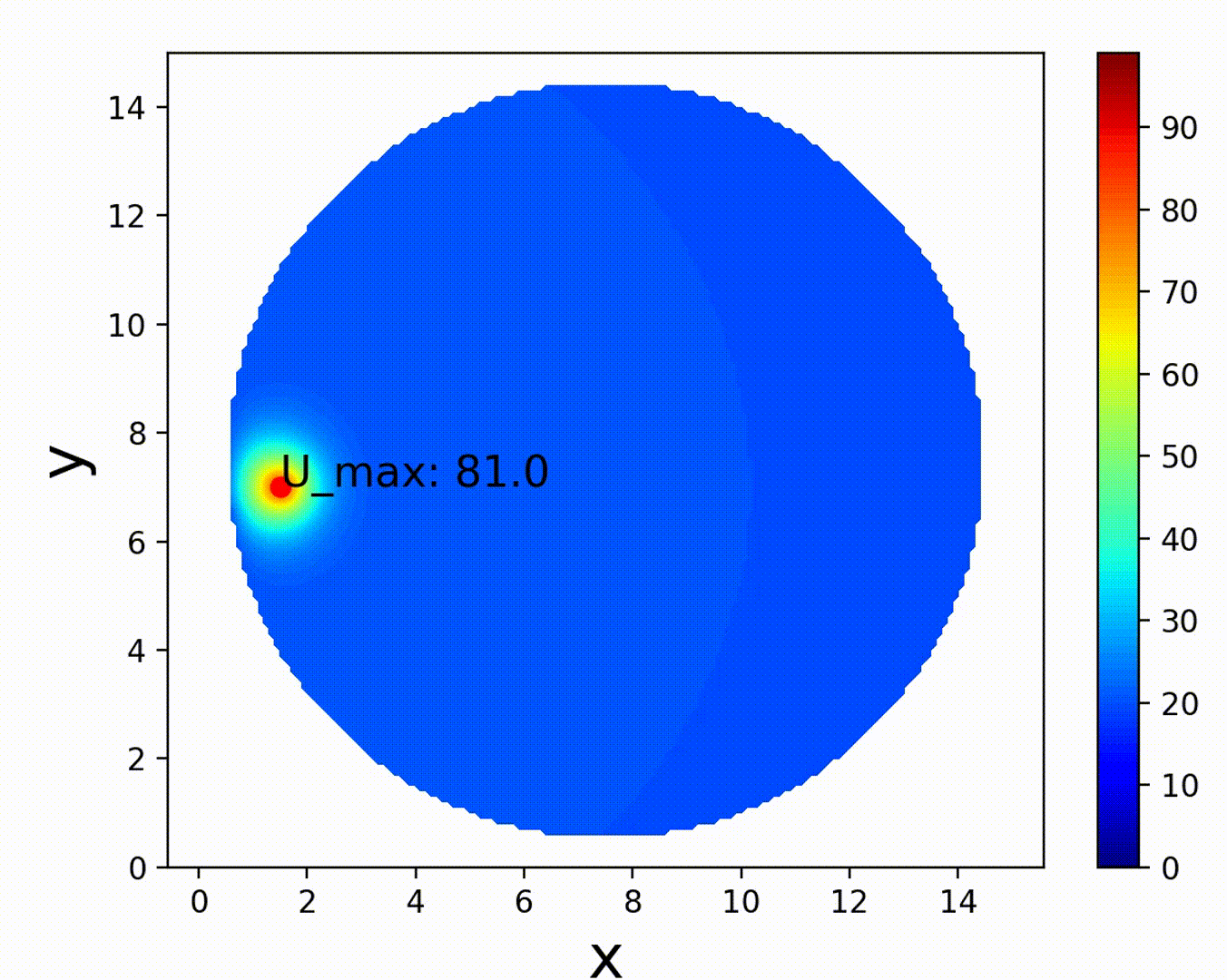不显示外部环境节点周期边界条件假设矩形计算区域代表的不是一个平面，而是一个首位相接的圆环外壁，那么这时候会出现一个十分有趣的周期边界。最左边的节点和最右边的节点实际上是相同的节点，这个周期边界该如何实现呢？代码上十分简单，只需要将二阶偏导系数矩阵的左下角和右上角的值置为1就可以了（以x方向为例），这样左右边界点的二阶导就可以由完整三项差分求得。# x方向二阶导系数矩阵A
A = (-2)*np.eye(Mx+1,k=0) + (1)*np.eye(Mx+1,k=-1) + (1)*np.eye(Mx+1,k=1)
A[0,-1] = A[-1,0] = 1

# solve boundary nodes 去掉X方向的热流边界约束
ch = 0.1e2
U[0,:] = (u_env + ch*U[1,:]/dy)/(1+ch/dy)
U[-1,:] = (u_env + ch*U[-2,:]/dy)/(1+ch/dy)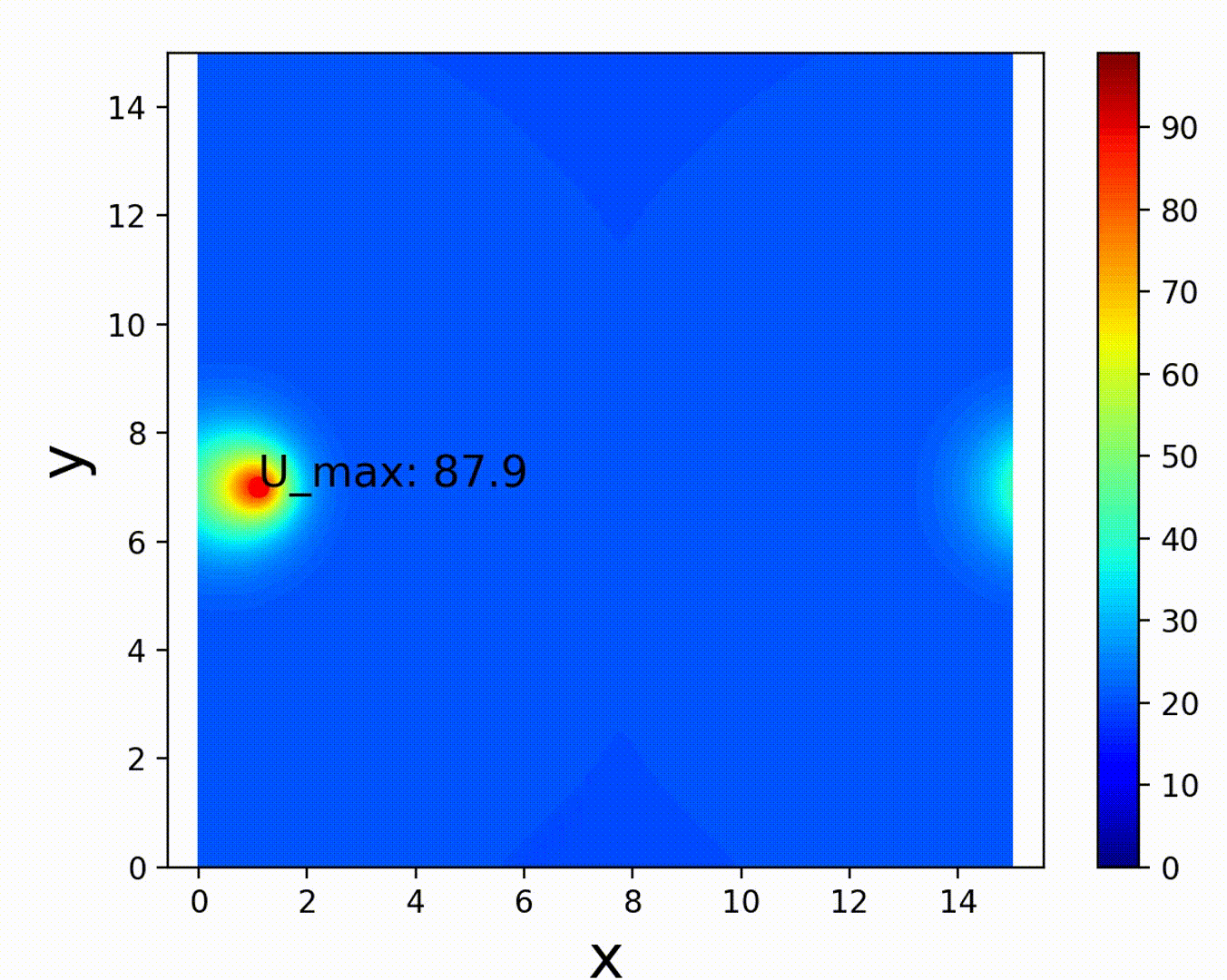周期边界，X方向连续可以看到x方向的左右边界是连续的、周期变化的，类似于圆环上的圆周切线方向。
展开全文• pizh12thu：Python数值优化：使用Euler法求解二维热传导方程1​zhuanlan.zhihu.com移动热源移动热源在焊接分析、3D增材制造等瞬态分析中十分常见。先前计算式中给了恒定常数的热源 ,由于Numpy的广播机制， 与矩阵...上一篇实践了简单的二维热传导抛物线方程求解，对于简单的热源和等温边界条件进行了模拟，下面来探究一些更有趣的现象。pizh12thu：Python数值优化：使用Euler法求解二维热传导方程1​zhuanlan.zhihu.com移动热源移动热源在焊接分析、3D增材制造等瞬态分析中十分常见。先前计算式中给了恒定常数的热源,由于Numpy的广播机制，与矩阵进行相加操作时会被扩展成和U形状相同的矩阵进行运算，为了控制热源的移动，需要对矩阵形式的热源进行操作。U = U + rx*np.dot(U,A) + ry*np.dot(B,U) + heat*ft在Python代码中实现，指定一个具有空间位置的热源import numpy as np
import time
import matplotlib.pyplot as plt

if __name__ == "__main__":
a = 1
D = np.array([0,15,0,15])
Mx = 150
My = 150
T,Tn = 20,5
Nt = 20000

dx = (D-D)/Mx
dy = (D-D)/My
dt = T/Nt
Frame = 100
fq = 10
u0 = 20
u_env = 20

### 初始化U、A、B矩阵，各点初始温度u0
U = u0*np.ones((My+1,Mx+1))
# x方向二阶导系数矩阵A
A = (-2)*np.eye(Mx+1,k=0) + (1)*np.eye(Mx+1,k=-1) + (1)*np.eye(Mx+1,k=1)
# y方向二阶导系数矩阵B
B = (-2)*np.eye(My+1,k=0) + (1)*np.eye(My+1,k=-1) + (1)*np.eye(My+1,k=1)

#用网格化映射矩阵坐标信息
x=np.linspace(D,D,U.shape)
y=np.linspace(D,D,U.shape)
X,Y = np.meshgrid(x,y)
x0,y0 = 0,7 #热源中心位置

### 按时间增量逐次计算
for k in range(Nt+1):
tt = k*dt
#热源沿着X方向移动
x0 += 2*dt
Qv = 80*fq*np.exp(-8*((X-x0)**2+(Y-y0)**2))
# solve inside nodes
U = U + rx*np.dot(U,A) + ry*np.dot(B,U) + Qv*dt
# solve boundary nodes
U[:,0] = U[:,-1] = u_env
U[0,:] = U[-1,:] = u_env 用等温云图显示一下计算结果        if k%Frame == 0:
end = time.time()
print('T = {:.3f} s  process time = {:.1f}'.format(tt,end-start))
showcontourf(U,D,vmax=120)

def showcontourf(mat,D,cmap=plt.cm.get_cmap('jet'),fsize=(12,12),vmin=0, vmax=100):
plt.clf()
levels = np.arange(vmin,vmax,1)
x=np.linspace(D,D,mat.shape)
y=np.linspace(D,D,mat.shape)
X,Y=np.meshgrid(x,y)
z_max = np.max(mat)
i_max,j_max = np.where(mat==z_max), np.where(mat==z_max)
show_max = "U_max: {:.1f}".format(z_max)
plt.plot(x[j_max],y[i_max],'go')
plt.contourf(X, Y, mat, 100, cmap = cmap, origin = 'lower', levels = levels)
plt.annotate(show_max,xy=(x[j_max],y[i_max]),xytext=(x[j_max],y[i_max]),fontsize=14)
plt.colorbar()
plt.xlabel('x', fontsize=20)
plt.ylabel('y', fontsize=20)
plt.axis('equal')
plt.draw()
plt.pause(0.001)
plt.clf()移动内热源的实现Neumann边界条件可以看到热源向右匀速移动的效果，但是左右边界上似乎有点奇怪，边界没有受到热源的影响。这是因为我们给定了边界上的节点温度，即Dirichlet边界条件，边界温度与环境温度始终相同，那么如何处理热流边界条件呢        # solve boundary nodes
U[:,0] = U[:,-1] = u_env
U[0,:] = U[-1,:] = u_env 热流边界条件是第二类边界条件即Neumann边界条件，给定边界节点处的一阶导（斜率）同样采用差分格式离散化，则有通常边界给定对流换热系数，这样就可以建立热流平衡方程，使边界点内外两边的温度梯度达到平衡，即这样边界温度值就可以根据环境温度和内部节点温度的值加权平均求得，代表导热系数，表示边界处的对流换热系数，系数就反映了热传导和对流的相对大小，也决定了边界节点温度更接近内部还是外部温度。假定环境温度保持不变，边界均为对流散热边界，则有将 solve boundary nodes 部分改为如下形式        # solve boundary nodes
ch = 0.1
U[:,0] = (u_env + ch*U[:,1]/dx)/(1+ch/dx)
U[:,-1] = (u_env + ch*U[:,-2]/dx)/(1+ch/dx)
U[0,:] = (u_env + ch*U[1,:]/dy)/(1+ch/dy)
U[-1,:] = (u_env + ch*U[-2,:]/dy)/(1+ch/dy)对流换热边界条件，边界热量流出速度下降如果，，那么边界节点温度将等于环境温度，与等温边界结果相同。如果，，那么将没有热量可以从边界流出，此时就形成了绝热边界条件，边界温度等于内部相邻节点温度，结果如下图绝热边界条件，等温线垂直于左右边界边界形状如果边界并非矩形，该如何用矩阵处理呢？关键在于确定矩阵中哪些节点是边界节点，可以使用Python中的Boolean逻辑矩阵。这里构造一个半径为7的圆形边界，用plt.imshow()来显示Boolean矩阵    x=np.linspace(D,D,U.shape)
y=np.linspace(D,D,U.shape)
X,Y = np.meshgrid(x,y)

Mat_e = (X-7.5)**2 + (Y-7.5)**2 >= 7**2
Mat_b = abs(((X-7.5)**2 + (Y-7.5)**2)**0.5 - 7) <= 0.5*min(dx,dy)
plt.imshow(Mat_b, cmap=plt.cm.get_cmap('jet'), origin = 'lower',vmin=0, vmax=1)
plt.show()Mat_e代表外部环境，Mat_b代表边界。离散的边界点不可能完全在解析曲线上，只要节点到边界曲线（圆周）的距离小于半个单元长度，就认为是边界节点。元素值为Ture的地方显示红色，False显示蓝色，边界节点外部节点求解边界时令外部节点温度等于环境温度，边界节点采用等效的热流边界，即以内部节点温度的最小值作为梯度参考，省略了计算法向的步骤。        # solve boundary nodes
ch = 0.1e6
U[Mat_e] = u_env
U[Mat_b] = (u_env + ch*np.min(U[~(Mat_e+Mat_b)])/dx)/(1+ch/dx)可以看到变成了圆形边界，但温差太小，分界不是很明显。为了使结果表达更清晰，可以让外部节点不显示呢，Python中可以通过将对应位置的点赋值为np.nan实现#在函数showcontourf增加下列语句，且多传入一个参数M_e
def showcontourf(mat,D,M_e,cmap=plt.cm.get_cmap('jet'),fsize=(12,12),vmin=0, vmax=100):
...
...
Z = mat.copy()
Z[M_e] = np.nan
plt.contourf(X, Y, Z, 100, cmap = cmap, origin = 'lower', levels = levels)

#在main函数中,传入外节点Boolean矩阵
showcontourf(U,D,Mat_e,vmax=100)注意这里需要将mat复制一份（mat.copy()）再进行赋值操作，否则将会改变原数组mat，后续计算无法进行。显示结果如下不显示外部环境节点周期边界条件假设矩形计算区域代表的不是一个平面，而是一个首位相接的圆环外壁，那么这时候会出现一个十分有趣的周期边界。最左边的节点和最右边的节点实际上是相同的节点，这个周期边界该如何实现呢？代码上十分简单，只需要将二阶偏导系数矩阵的左下角和右上角的值置为1就可以了（以x方向为例），这样左右边界点的二阶导就可以由完整三项差分求得。# x方向二阶导系数矩阵A
A = (-2)*np.eye(Mx+1,k=0) + (1)*np.eye(Mx+1,k=-1) + (1)*np.eye(Mx+1,k=1)
A[0,-1] = A[-1,0] = 1

# solve boundary nodes 去掉X方向的热流边界约束
ch = 0.1e2
U[0,:] = (u_env + ch*U[1,:]/dy)/(1+ch/dy)
U[-1,:] = (u_env + ch*U[-2,:]/dy)/(1+ch/dy)周期边界，X方向连续可以看到x方向的左右边界是连续的、周期变化的，类似于圆环上的圆周切线方向。
展开全文• 热传导方程一维问题问题背景问题抽象离散方法1 向前差分迭代格式初值设定取参数C语言实现fortran实现画图 hexo博客为啥用不了了，将就着先用这个博客吧 一维问题 问题背景 摘抄自网络 ：在距离为L的两个半无限长壁...
热传导方程一维问题问题背景问题抽象离散方法1 向前差分迭代格式初值设定取参数C语言实现fortran实现画图

hexo博客为啥用不了了，将就着先用这个博客吧

一维问题
问题背景
摘抄自网络 ：在距离为L的两个半无限长壁面之间有传热的流体。假设整个流场初始时刻具有温度T=T1(常数，本题中取10摄氏度)，并处于平衡状态(即：初始时刻壁面和流体的温度都为10摄氏度)。两个壁面的初始温度Tw1=Tw2=T1（本题中即为10摄氏度）。现假设在t=0时刻，右边的壁面温度突然增加到Tw2=T2并保持在T2（本题中取T2为20摄氏度），而左边的壁面温度保持在Tw1=T1（即10摄氏度）。求时间t1、t2和时间趋于无穷时壁面间流体温度的分布？
问题抽象
$u_t=\alpha\cdot \frac{\partial^2u}{\partial x^2} \\ 初值：u(x,0)=u_0(x)\\ 边值：u(0,t)=\mu_0(t),u(l,t)=\mu_1(t)$
离散方法
1 向前差分
$\frac{u(x_i,t+\Delta t)-u(x_i,t)}{\Delta t}=\alpha\cdot\frac{u(x_{i+1},t)-2u(x_{i},t)+u(x_{i-1},t)}{\Delta x^2}$
迭代格式
$u(x_i,t+\Delta t)=ru(x_{i+1},t)+(1-2r)u(x_i,t)+u(x_{i-1},t)$
其中$r=\frac{a\Delta t}{(\Delta x)^2}$
初值设定
$T_1=10\\ T_2=20\\ L=0.2m\\ t=10s$
取参数
$dx=0.01\\ dt=0.001$
C语言实现
#include <stdio.h>
#include <stdlib.h>
#include<math.h>
int main()
{
float dx,dt,t,L=0.2,a,b,alpha=1.42857*10e-3;
/*dx表示delta(x)，dt表示delta(t)，alpha为热扩散率。由于数组大小随输入值而变化，所以取得比较大*/
int i,n1,n2,n=0,total=1;
FILE *F;//文件指针
F=fopen("data.txt","w");
dx=0.01;
dt=0.001;
t=10;
n1=(int)(L/dx);
n2=(int)(t/dt);
//printf("n1=%d  n2=%d\n",n1,n2);
for(i=0;i<n1;i++){     /*初始赋值*/
if(i<n1-1){
a[i]=10;
}
else a[i]=20;
fprintf(F,"%8.4f",a[i]);//写入t=0时刻的温度
}
fprintf(F,"\n");    //换行
while(n<n2){    /*时间到了，停止循环*/
for(i=0;i<n1;i++){
/*计算*/
if(i==0){
b[i]=10;
a[i]=b[i];
}     /*表示左壁面的温度始终保持在10摄氏度*/
else if(i==n1-1){
b[i]=20;
a[i]=b[i];
}     /*表示右壁面的温度始终保持在20摄氏度*/
else{
b[i]=a[i]+alpha*dt*(a[i+1]-2*a[i]+a[i-1])/(dx*dx);   /*差分方程的表达式*/
a[i]=b[i];
}
n++;
fprintf(F,"%8.4f",a[i]);
//printf("%8.4f",a[i]);
if(total%n1==0){             /*输出data文件*/
//printf("\n");
fprintf(F,"\n");
}
total++;
}
}
fclose(F);
return 0;
}


输出结果为data.txt
fortran实现
program f1
implicit none
integer::i,n1,n2,n,total,coun
real::a(100000),b(100000)
real::dx,dt,t,L,alpha
open(unit=1,file='data-f.txt')  !打开文件
n=1
total=0
dx=0.01
dt=0.001
t=10 !时间
L=0.2   !长度
alpha=1.42857*10e-3
n1=int(L/dx)
n2=int(t/dt)
do coun=1,n1        !类似于C语言的for循环
if (coun<n1) then
a(coun)=10      !赋初始值
else
a(coun)=20
end if
end do
do while(n<n2)!时间限制
do i=1,n1
if (i==1) then
b(i)=10         !表示左壁面的温度始终保持在10摄氏度
a(i)=b(i)
else if(i==n1) then     !表示右壁面的温度始终保持在20摄氏度
b(i)=20
a(i)=b(i)
else                    !其他情况需要用差分方程计算
b(i)=a(i)+alpha*dt*(a(i+1)-2*a(i)+a(i-1))/(dx*dx)
a(i)=b(i)
end if
write(1,"(F8.4)",advance='no')a(i)!advancce=n0使得每次读写结束，位置不会自动向下移动一行
if(mod(n,n1)==0) then
write(1,*)                      !每一行够n1个之后换行
end if
n=n+1
end do
end do
do coun=1,n1
write(*,"(F8.4)")a(coun) !控制台输出t=10s的温度分布
end do
close(unit=1)                   !关闭文件
end program

数据储存在文件data-f.txt中
画图
想画随时间变化的动图，不知道怎么画


展开全文算法
• a为方程中的系数b，可任取 I = 1 / h ; N = maxt / deltat ; sol = zeros ( fix ( I + 1 ) , fix ( N + 1 ) ) ; mu = a * deltat / h ^ 2 ; vetx = [ 0 : h : 1 ] ; veti = ones ( I - 1 , ...matlab
• 一维非定常热传导方程FTCS格式Fortran源程序Fortran